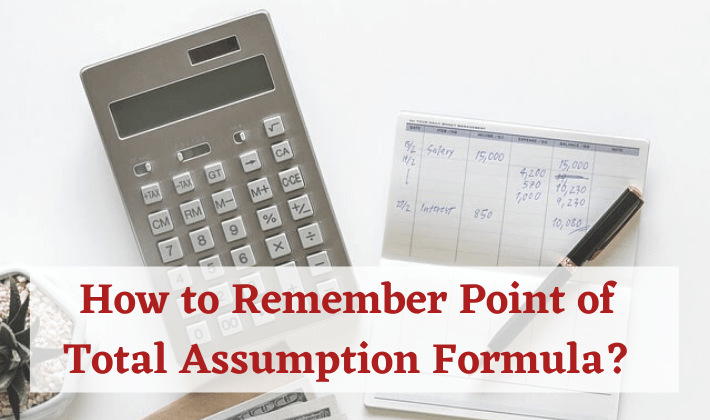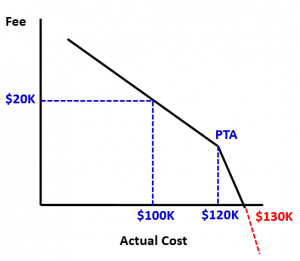## Point of Total Assumption Formula in Fixed Price Incentive Fee Contract

How do you remember any formula?

Simple! Understand the concept you will not need to remember the formula.

I heard about Point of Total Assumption when I was preparing for PMP® Exam. At the beginning, it sounded very funny. One of the meanings of word “Assumption” is “the act of taking possession of something” e.g. “Assuming the power”. Although this is a more popular meaning, but there are other connotations of this word. It also means “the act of taking over another person’s debts or obligations”. In Project Management, the word refers to “taking over Extra Costs”. More on that later…

Note: The meaning of word Assumption is different in Project Assumptions.

I have written two articles on Fixed Price Incentive Fee Contract (FPIF). This is the third and concluding article on the subject. I gave a general description of FPIF Contract in my first article. In the second article, I talked about 5 mathematical formulas of FPIF Contract. In this article, I will talk about Point of Total Assumption Formula.

### What is Point of Total Assumption?

In the previous article, we discussed cost driven FPIF Contract. We determined how additional expenditure above the Target Cost (TC) is shared between the buyer and the seller. The share of the additional cost is governed by the agreed upon Share Ratio (SR). But we also saw that Buyer’s liability is limited only up to the Ceiling Price (CP). If the cost goes above a particular limit, then the seller has to bear the full cost above that limit. This limit is called Point of Total Assumption (PTA).

The Point of Total Assumption is the point above which the seller starts assuming the cost of the contracted work.

Let us understand this through an example.

#### Example

Let us go consider the example from the previous post.

Target Cost (TC) = 100K

Target Fee (TF) = \$20K

Ceiling Price (CP) = \$130K

Share Ratio = 50:50 (both the buyer and the seller get 50% of the Cost Variance)

Target Price (TP) = \$100K + \$20K = \$120K

Let us look at a particular scenario when Actual Cost is \$120K. Let us calculate the Actual Price.

Actual Cost (AC) = \$120K

Referring to the Formula II from the previous post

Cost Variance (CV) = \$100K – \$120K = -\$20K

The seller has spent \$20K more than the Target Cost. The extra cost will be divided between the buyer and the seller in the ratio of 50:50. Referring to the Formula II from the previous post.

Seller’s Share = (-20)*50% = -\$10K

Referring to Formula V from the previous post

Fee = \$20K – \$10K = \$10K

Referring to Formula I from the previous post

Price = \$120K + \$10K = \$130K

The buyer will pay \$130K to the Seller, which is also the Ceiling Price. The price is capped at \$130K. So, if the seller spends anything more than \$120K,  the extra cost will have to be borne by the seller. For our example, PTA is \$120K.### Formula VI – Point of Total Assumption Formula

PTA = (Ceiling Price – Target Price)/Buyer’s Share Ratio + Target Cost

Let us first derive this formula. We will use the following abbreviations for the ensuing discussion

• Ceiling Price – CP
• Target Price – TP
• Target Cost – TC
• Target Fee – TF
• Actual Cost – AC
• Seller’s Share Ratio – SR
• Buyer’s Share Ratio – BR

Going by the definition and above example, we already know two things. At PTA

• AC will be equal to PTA
• the buyer will pay CP to the seller

Referring to Formula I from the previous post

CP = PTA + Fee

Referring to Formula II, IV & V from the previous post

CP = PTA + [TF + (TC – AC) *SR]

In our case, AC is PTA. Replacing AC with PTA we get

CP = PTA + [TF + (TC – PTA)*SR]

The total of Buyer’s and Seller’s Share Ratio is 1. So we can say SR = 1 – BR. Replacing this in the above equation

CP = PTA + [TF + (TC – PTA)*(1-BR)]

CP = PTA + TF + TC – TC*BR – PTA + PTA*BR

CP = (PTA*BR – TC*BR) + (TF + TC)

CP = (PTA – TC)*BR + TP

Solving the above equation for PTA, we get our final PTA formula

PTA = (CP – TP)/BR + TC

PTA formula also gives us \$120K as the answer.

### Over To You

Was the explanation and derivation of Point Of Total Assumption formula helpful? Do you think you would be able to remember the formula now? Please leave a comment if you have a question.

#### Related Articles

Formal Explanation Of Different Contract Types For PMP Exam

Explanation of Different Contract Types Using Examples

### PMP Exam Formulas

I have also compiled a PMP Formulas Cheat Sheet. It contains 45 formulas and 57 abbrviations. It will help you in your exam prep. It is the best and most comprehensive cheat sheet based on the PMBOK Guide 6th edition. You can download it free of cost for your studies.

If you are looking beyond a cheat sheet, then I would suggest you to buy detailed PMP Exam Formula Study Guide by Cornelius Fichtner. It contains detailed explanations of all the formulas along with examples and 105 practice questions.

Disclosure: This article contains affiliate links - it means that, if you buy from any of these links, then I will receive a small commission that would help me in maintaining this blog for free. However, for you, there is no extra cost. I recommend only those products that I believe will definitely help the certification aspirants.

## One Comment

1. upendrav says:

Very different and thorough. Thanks.